# .css-4zleql{display:block;}std::steam# 100 Days Of ML Code — Day 067

Jehoshaphat I. Abu
·Sep 14, 2018·

## Recap From Day 066

Day 066, we looked at how Gesture Variation Follower works. You can catch up using the link below. 100 Days Of ML Code — Day 066 Recap From Day 065medium.com

Today, we’ll continue from where we left off in day 066

## Working with time

### How Gesture Variation Follower works continued.

To answer the question from yesterday how do we infer? So, a straightforward way will be to take the incoming observation, the current X, Y value from the mouse and to compuderically the relative size, speed and so on through a clever formula. This is however not feasible in practice because the observations, that is to say, the captured data by the mouse or other motion capture system, are noisy. Which will make such a calculus highly not accurate. Instead, GVF will proceed by sampling.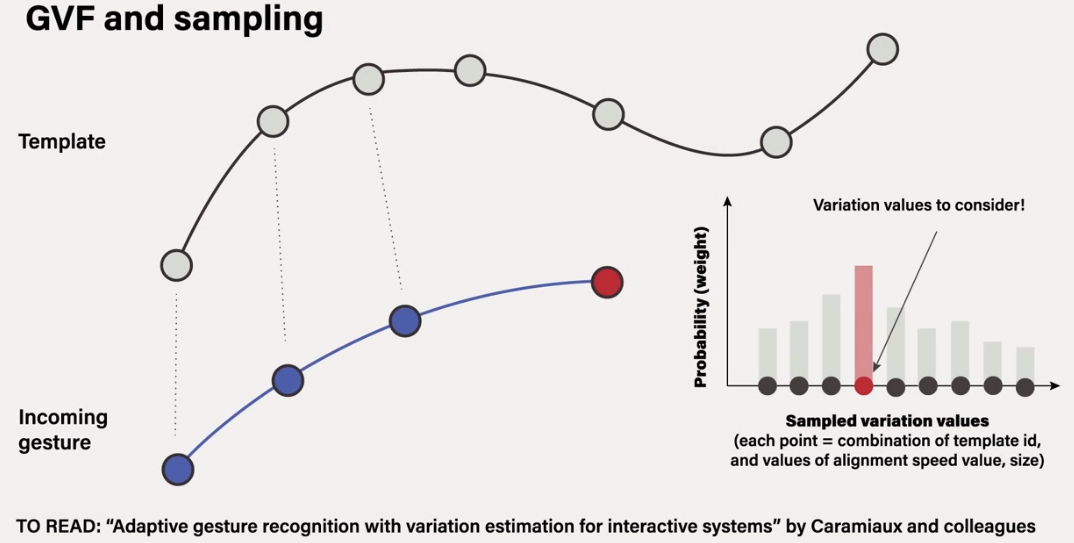Source

Sampling means that instead of considering one potential value per element to estimate, that is to say, one relative side value, one relative speed value, one gesture recognized and so on, GVF will consider hundreds of combinations of potential values simultaneously.

Of course they are not all good, actually only one will be the closest to the true estimations that we want. So the challenge is to select the best one among all of them. To do so the algorithm will take the incoming observation in order to weight each combination of potential value according to their likelihood. A weight close to one means that the combination is good. A weight close to zero means that the combination of values shouldn’t be taken into account.

To clarify let’s explicit one specific iteration of the algorithm for a particular combination of estimated values. let’s suppose that this particular combination is gesture index equals two, so a square for instance. Progressions bar equals 0.1, size 0.8, speed 1.2. So first the algorithm gets the gesture templates given by index two which is the square. Remember that the gesture template is a sequence of features.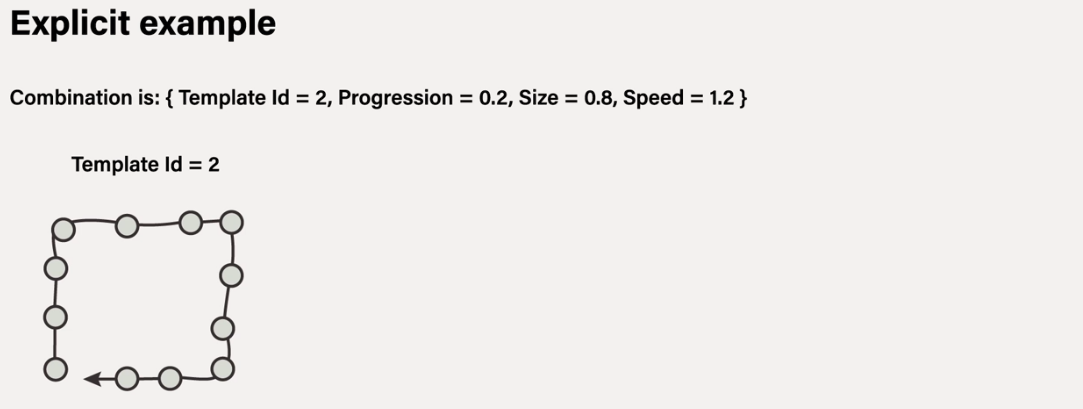Source

The algorithm then picks in the sequence of features the point at the specific time given by the progression value, 0.2 as seen in the image below. So exactly 20% of the template.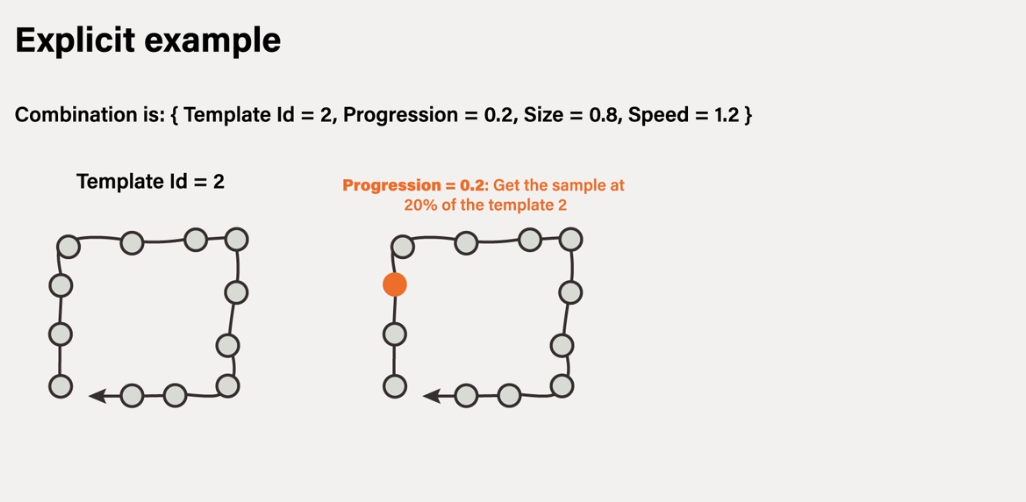Source

So far this gives us an X, Y value corresponding to the value at 20% of gesture two. Then we scale these values by the estimated size. So we have a new coordinate which is the scale value. Which gives us at the end of the process a transformed version of the X, Y value picked in the template.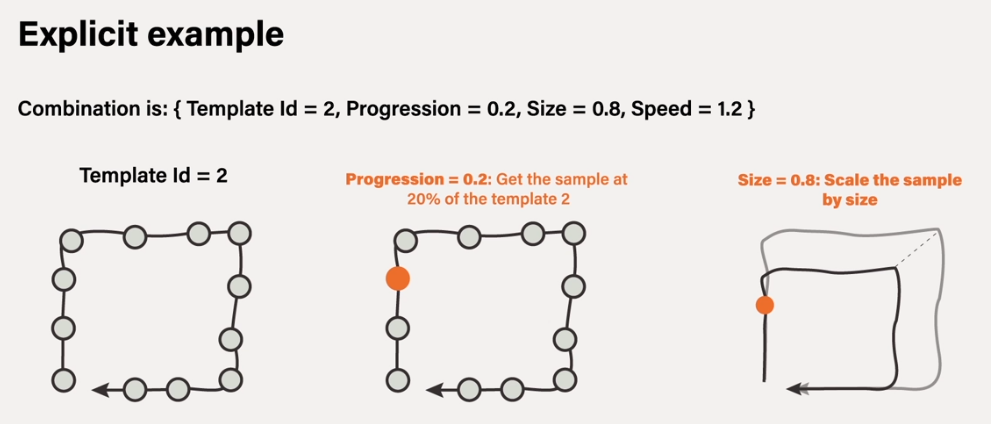Source

The next step is to compute the likelihood of the incoming observation given the transform value computed above. This likelihood value will give the weight for this current estimation. To do so we compute first a Euclidean distance between this value and the incoming observation. The resulting value gives an idea of the goodness of fit of our estimation.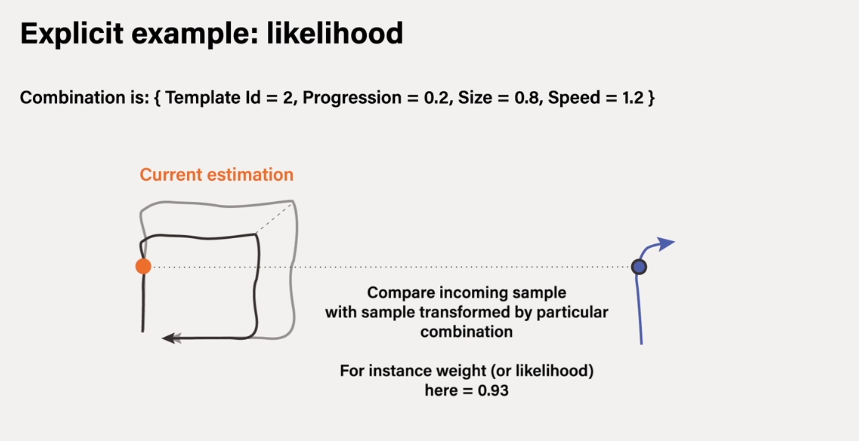Source

If the value is close to zero, we have a good estimate. Otherwise no. In the end, this value is made in a probability range between zero and one where one means good and this is made in order to be considered as likelihood.

That’s all for day 067. I hope you found this informative. Thank you for taking time out of your schedule and allowing me to be your guide on this journey. And until next time, be legendary.

References